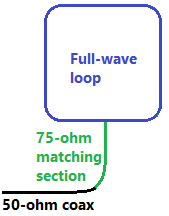# Full-Wave Loop Antenna Length Calculator

Full-wave loop antennas can be large at lower frequencies, but they are quieter than dipoles and have more gain broadside to the plane of the loop. A circular loop is ideal, but squares, triangles, and other shapes can work well; just make the area enclosed by the loop as big as you can.

You can use a full-wave loop on frequencies higher than design frequency as well (for example, using a 7 MHz loop on 21 MHz), but the pattern will be different.Full-wave loop fed with an impedance-matching transfromer made from a quarter-wave section of 75-ohm coaxial cable

To use the calculator, enter the desired operating frequency in megahertz.

Frequency in MHz:

### Length of full-wave loop:

The calculated length is approximate. In practice it's best to make the antenna a little longer than the calculated value and then trim it to get the best SWR value.

### Feedpoint impedance and coaxial matching section

The feedpoint impedance at the design frequency is about 100 ohms, so full wave loops are often fed with an impedance-matching section made from a piece of 75-ohm coxial cable one quarter wavelength long (shortened to compensate for the velocity factor of the cable).

Length of an impedance-matching section with 75-ohm cable with a velocity factor of 0.66 (polyethylene dielectric):

Length of an impedance matching section made with 75-ohm cable with a cable velocity factor of 0.80 (foam dielectric):

### Formulas

The formulas for calculating the approximate length of a full-wave loop antenna are:

• Full-wave loop in feet = 1005 / frequency in MHz
• Full-wave in meters = 306 / frequency in MHz

The formulas for calculating the length of an impedance matching section are:

• Quarter-wave matching section length in feet = 246 * cable's velocity factor / frequency in MHz
• Quarter-wave matching section length in meters = 75 * cable's velocity factor / frequency in MHz

The velocity factor for polyethylene coax is usually 0.66. For foam-dielectric cable, it's usually 0.8.

The antenna can be connected by other means, of course, including parallel-conductor lines, which work best when using the antenna on multiple bands.

### References

The ARRL Antenna Book for Radio Communications